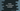# What is elvis operator in Kotlin# What is elvis operator in Kotlin :

Elvis operator is used to removing null pointer exception in Kotlin. We can use it to check if any variable is null or not and it allows us to use one default value if the value is null. The syntax of elvis operator is ?:.

``val r = x ?: y``

Here, the value of r will be x if x is not null. If it is null, it will be y. Note that we can use any expression to the left and right-hand side of the elvis operator. The right-hand expression will be evaluated only if the left side is null.

## Example :

``````fun main(args: Array) {
println(getLength("hello"))
println(getLength(null))
}

fun getLength(input: String?) : Int{
return input?.length ?: -1
}``````

This program will print the below output :

``````5
-1``````

As you can see that the first call to getLength with hello as parameter printed its length 5. But if we are passing a null value as the parameter to the function, it will return -1.

## Using throw and return :

We can also use throw and return to the right of an elvis operator to return a specific value or to throw an exception. For example, we can modify the above example as below :

``````fun main(args: Array) {
println(getLength("hello"))
println(getLength(null))
}

fun getLength(input: String?) : Int{
val length = input?.length ?: return -1
return length
}``````

The output of the program will be the same as the previous example. The only advantage of this modification is that if you are doing any operation with the length variable after it is calculated, we can avoid these calculations and return -1 if the input string is null. Similarly, we can also throw an exception if the left side of the elvis operator failed :

``````fun main(args: Array) {
println(getLength("hello"))
println(getLength(null))
}

fun getLength(input: String?) : Int{
val length = input?.length ?: throw IllegalArgumentException("Null input")
return length
}``````

It will throw one exception :

``````5
Exception in thread "main" java.lang.IllegalArgumentException: Null input
at ExampleKt.getLength(example.kt:7)
at ExampleKt.main(example.kt:3)``````

### Conclusion :

Elvis is very useful to remove null pointer exception with only one line of code. Try to run the examples we have shown above and drop one comment below if you have any queries.

You might also like :